# 04-Graphing and analyzing data¶

## 4.1 physica¶

physica is a CLI-driven data plotting and analysis program that is using full MINUIT fitting package to combine outstanding data analysis capabilities with publication-quality output generation. It's been used at Brock for more than 20 years, and is embedded into all of our Y1 lab experiments (in a simplified form). It exists in both a tcl-wrapped physicalab and an online variants, the latter with a brief tutorial and an extensive online help facility.

physica has a flexible macro language, Fortran-like in its syntax.

Below is a brief introduction to its basic functionality:

In :
%%file graph2d.pcm
x=[1:8]
y=[0.05;0.10;0.14;0.19;0.25;0.30;0.34;0.40]
dy=[0.02;0.07;0.01;0.04;0.05;0.07;0.02;0.04]

label\x Time, s'
label\y Distance, m'
set pchar -10
graph x,y,dy

scalar\vary a
fit y=a*x
fit\update f

set pchar 0
graph\noaxes x,f

hardcopy PNG
quit

Overwriting graph2d.pcm


We can execute a non-interactive version of physica and pass this set of commands to it.

In :
%%bash
physica-www < graph2d.pcm > graph2d.png


There are multiple ways of displaying the resulting output within the notebook. The first two require the use of a markdown-type cell, which is quick and seamless, but unfortunately, may not update properly since the browser caching cannot be easily turned off, so even if the figure changes - you modify and re-run the script - the copy shown in the notebook may well be the stale cached version.In :
%%html
<img src="graph2d.png">A more robust way is to execute some native python code which bypasses the caching by the browser.

In :
from IPython.display import display, Image
display(Image('graph2d.png'))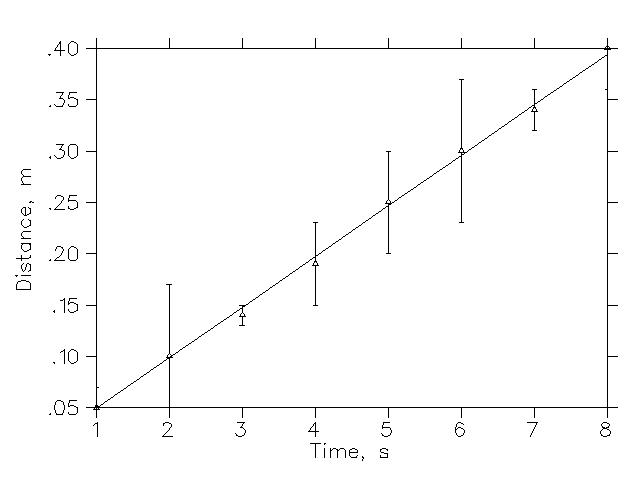Here's a slightly more elaborate version of the same macro, with slightly refined annotation and colour use.

In :
%%file graph2d.pcm
! graph2d.pcm
!   - a simple data plot, with a fit to a linear dependence
!
! By:        edward.sternin@brocku.ca
! Completed: 2018.02
!

x=[1:8]
y=[0.05;0.10;0.14;0.19;0.25;0.30;0.34;0.40]
dy=[0.02;0.07;0.01;0.04;0.05;0.07;0.02;0.04]

scales 0.5 8.5 7 0 0.45 4
set
xvmin 1
xvmax 8
yvmax 0.4
font triumf.2
%charsz 2
yleadz 1

scalar\vary a
fit y=a*x
fit\update f

label\x Time, s'
label\y Distance, m'

set pchar -10
graph x,y,dy

set pchar 0
set colour 3
graph\noaxes x,f

hardcopy PNG
quit

Overwriting graph2d.pcm

In :
%%bash
physica-www < graph2d.pcm > graph2d.png

In :
from IPython.display import display, Image
display(Image('graph2d.png'))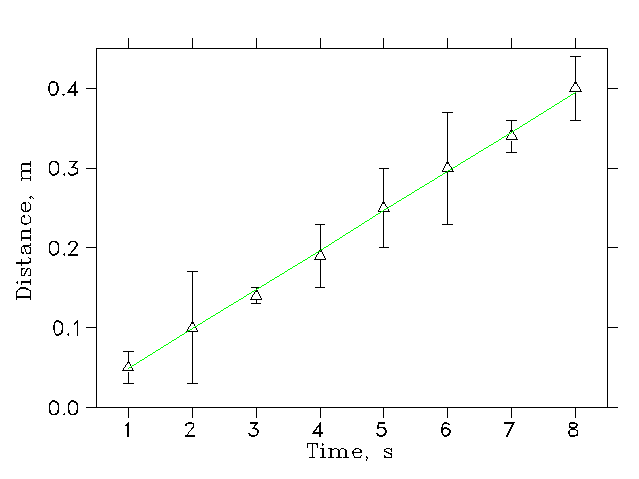## 4.2 gnuplot¶

gnuplot is one of the most widely used programs, and is available for a wide variety of platforms (even for Android!). It has a quick learning curve for simple graphs, but anything elaborate or requiring some serious computations, even if possible, gets to be quite difficult quickly. The current version of gnuplot is 5.2 and there is an excellent reference book:

In :
# This loads the gnuplot kernel extension, for embedded gnuplot use
%load_ext gnuplot_kernel
%gnuplot inline pngcairo size 800,1200 font "Palatino,16"

In :
%%file VI.dat
#  V   I,mA
0   0.468
1   0.405
2   0.342
3   0.279
4   0.216
5   0.153
6   0.090
6.4 0.064

Overwriting VI.dat

In :
%%gnuplot
## a sample script to demonstrate some more advanced features of gnuplot
## By edward.sternin@brocku.ca
## 2018.02

## outside of the jupyter notebook, can use these lines instead of a separate data file,
## and then use $DATA instead of every occurrence of 'VI.dat' below. It's a limitation of ## jupyter, and not of gnuplot. #$DATA << EOD
##  V   I,mA
#  0   0.468
#  1   0.405
#  2   0.342
#  3   0.279
#  4   0.216
#  5   0.153
#  6   0.090
#  6.4 0.064
#EOD

# scan through the data file
stats 'VI.dat'
# a few examples of useful values:
#x_max=STATS_max_x
#y_min=STATS_min_y
#Npts=STATS_records
#  etc.

## fit the data
set dummy v
P(v)=a*(v-Vo)**2+b
Vo=4
a=-15
b=0.85
# fit does NOT like zero starting values for any parameters, so do not skip the previous lines
fit P(v) 'VI.dat' using ($1):($1)*($2) via Vo,a,b # two rows, one column is the layout of outputs for our multiple "plot ..." commands set multiplot layout 2,1 ## upper frame, P vs. V set xlabel 'V, Volts' set ylabel 'Power P=VI, mW' plot[0:6.5] [0.:1.2] 'VI.dat' using ($1):($1)*($2) with points pt 6 ps 1.25 t 'measured P=VI',\
[STATS_min_x:STATS_max_x] P(v) t sprintf("fit: %.2f(V-%.2f)^2+%.2f",a,Vo,b),\
'+' using (Vo):(-0.4):(0):(0.4+b) with vectors t sprintf("P_{max} at V=%.2f V",Vo)

## lower frame, R vs. V
Ro=Vo**2/b
set xlabel 'V, Volts'
set ylabel 'Resistance R=V/I, {/Symbol W}'
plot [0:6.5] [0:60] 'VI.dat' using ($1):($1)/($2) with points pt 6 ps 1.25 t 'measured R=V/I',\ [STATS_min_x:STATS_max_x] (v<=5.3 ? v**2/P(v) : NaN ) t 'fit: V^2/P',\ '+' using (Vo):(60):(0):(-60+Ro) with vectors ls 3 t sprintf("P_{max} at V=%.2f V",Vo),\ '+' using (Vo):(Ro):(-Vo):(0) with vectors ls 3 t sprintf("at P_{max}, R=%.2f {/Symbol W}",Ro) unset multiplot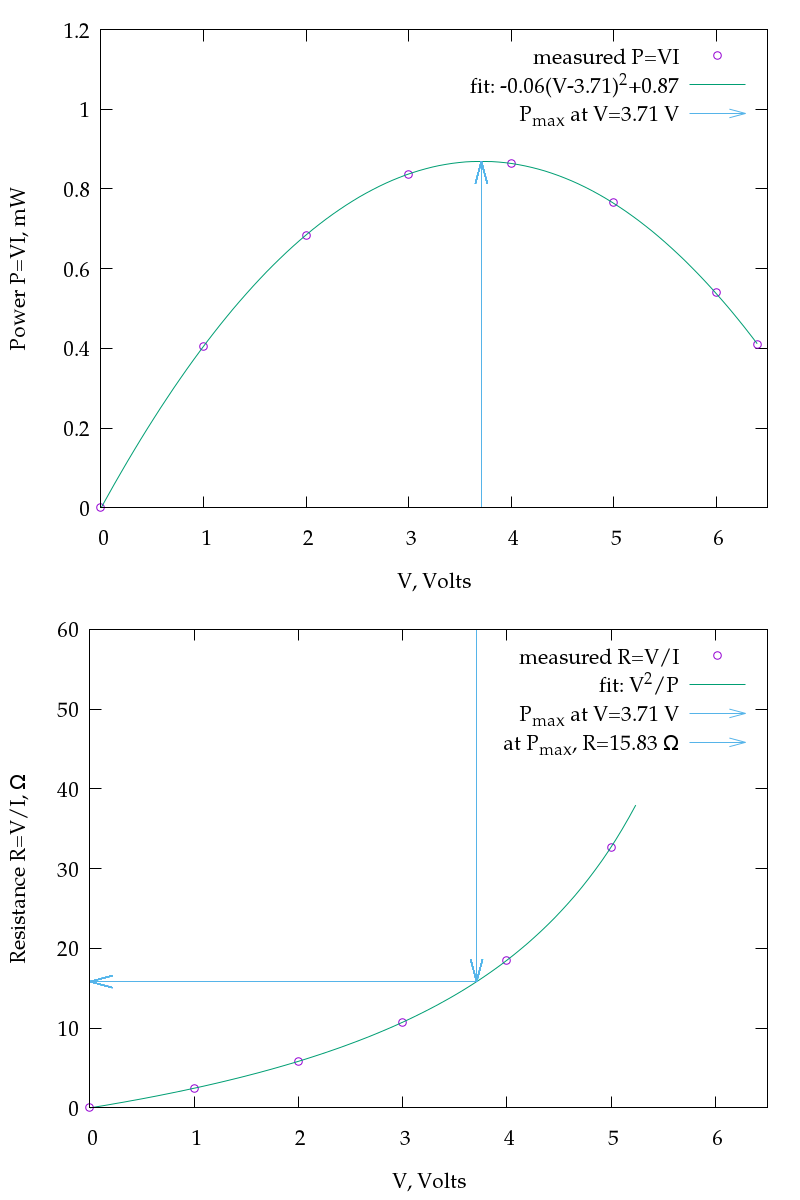## a sample script to demonstrate some more advanced features of gnuplot ## By edward.sternin@brocku.ca ## 2018.02 ## outside of the jupyter notebook, can use these lines instead of a separate data file, ## and then use$DATA instead of every occurrence of 'VI.dat' below.  It's a limitation of
## jupyter, and not of gnuplot.

#$DATA << EOD ## V I,mA # 0 0.468 # 1 0.405 # 2 0.342 # 3 0.279 # 4 0.216 # 5 0.153 # 6 0.090 # 6.4 0.064 #EOD # scan through the data file stats 'VI.dat' * FILE: Records: 8 Out of range: 0 Invalid: 0 Column headers: 0 Blank: 0 Data Blocks: 1 * COLUMNS: Mean: 3.4250 0.2521 Std Dev: 2.1827 0.1376 Sample StdDev: 2.3335 0.1472 Skewness: -0.1191 0.1168 Kurtosis: 1.6791 1.6801 Avg Dev: 1.9250 0.1214 Sum: 27.4000 2.0170 Sum Sq.: 131.9600 0.6601 Mean Err.: 0.7717 0.0487 Std Dev Err.: 0.5457 0.0344 Skewness Err.: 0.8660 0.8660 Kurtosis Err.: 1.7321 1.7321 Minimum: 0.0000  0.0640  Maximum: 6.4000  0.4680  Quartile: 1.5000 0.1215 Median: 3.5000 0.2475 Quartile: 5.5000 0.3735 Linear Model: y = -0.06306 x + 0.4681 Slope: -0.06306 +- 4.241e-05 Intercept: 0.4681 +- 0.0001723 Correlation: r = -1 Sum xy: 4.505 # a few examples of useful values: #x_max=STATS_max_x #y_min=STATS_min_y #Npts=STATS_records # etc. ## fit the data set dummy v P(v)=a*(v-Vo)**2+b Vo=4 a=-15 b=0.85 # fit does NOT like zero starting values for any parameters, so do not skip the previous lines fit P(v) 'VI.dat' using ($1):($1)*($2) via Vo,a,b
iter      chisq       delta/lim  lambda   Vo            a             b
0 9.0276894603e+04   0.00e+00  1.68e+02    4.000000e+00  -1.500000e+01   8.500000e-01
1 7.7401581630e+03  -1.07e+06  1.68e+01    3.709145e+00  -4.870474e+00   8.539867e-01
2 1.4393124873e-01  -5.38e+09  1.68e+00    3.706914e+00  -8.241128e-02   8.560346e-01
3 1.8474236621e-04  -7.78e+07  1.68e-01    3.711613e+00  -6.232464e-02   8.621812e-01
4 1.1747302856e-05  -1.47e+06  1.68e-02    3.709792e+00  -6.319534e-02   8.692526e-01
5 1.1723193296e-05  -2.06e+02  1.68e-03    3.709757e+00  -6.320607e-02   8.693376e-01
6 1.1723193294e-05  -1.18e-05  1.68e-04    3.709757e+00  -6.320607e-02   8.693376e-01
iter      chisq       delta/lim  lambda   Vo            a             b

After 6 iterations the fit converged.
final sum of squares of residuals : 1.17232e-05
rel. change during last iteration : -1.18416e-10

degrees of freedom    (FIT_NDF)                        : 5
rms of residuals      (FIT_STDFIT) = sqrt(WSSR/ndf)    : 0.00153122
variance of residuals (reduced chisquare) = WSSR/ndf   : 2.34464e-06

Final set of parameters            Asymptotic Standard Error
=======================            ==========================
Vo              = 3.70976          +/- 0.002165     (0.05835%)
a               = -0.0632061       +/- 0.0001394    (0.2205%)
b               = 0.869338         +/- 0.0008429    (0.09696%)

correlation matrix of the fit parameters:
Vo     a      b
Vo              1.000
a               0.422  1.000
b              -0.245 -0.762  1.000

# two rows, one column is the layout of outputs for our multiple "plot ..." commands
set output '/tmp/gnuplot-inline-1520556393.8152409.748898697384.png'
set multiplot layout  2,1

## upper frame, P vs. V
set xlabel 'V, Volts'
set ylabel 'Power P=VI, mW'
plot[0:6.5] [0.:1.2] 'VI.dat' using ($1):($1)*($2) with points pt 6 ps 1.25 t 'measured P=VI', [STATS_min_x:STATS_max_x] P(v) t sprintf("fit: %.2f(V-%.2f)^2+%.2f",a,Vo,b), '+' using (Vo):(-0.4):(0):(0.4+b) with vectors t sprintf("P_{max} at V=%.2f V",Vo) ## lower frame, R vs. V Ro=Vo**2/b set xlabel 'V, Volts' set ylabel 'Resistance R=V/I, {/Symbol W}' plot [0:6.5] [0:60] 'VI.dat' using ($1):($1)/($2) with points pt 6 ps 1.25 t 'measured R=V/I', [STATS_min_x:STATS_max_x] (v<=5.3 ? v**2/P(v) : NaN ) t 'fit: V^2/P', '+' using (Vo):(60):(0):(-60+Ro) with vectors ls 3 t sprintf("P_{max} at V=%.2f V",Vo), '+' using (Vo):(Ro):(-Vo):(0) with vectors ls 3 t sprintf("at P_{max}, R=%.2f {/Symbol W}",Ro)

unset multiplot
unset output

gnuplot also has some programming ability. Here's an example of a recursively-defined function. It plots a truncated FT decomposition of a square wave, to an arbitrary order of truncation:

In :
%gnuplot inline pngcairo size 640,480 font "Palatino,12"

In :
%%gnuplot
# for a clean start, as gnuplot may remember things from previous scripts
reset

# set parameters controlling the appearance of the graphs
set xrange [-2.1*pi:2.1*pi]
set yrange [-1.4:1.4]
set samples 2001
set key below
set zeroaxis

# define the Fourier series' expansion iteratively: sin(x)+sin(3x)/3+sin(5x)/5+...
f(x,n) = (n==1) ? (4/pi)*sin(x) : f(x,n-1) + (4/pi)*sin((2*n-1)*x)/(2*n-1)

# plot the original square wave, and a couple of interesting approximations
plot sgn(sin(x)) t 'square wave' lw 2,\
f(x,3)  t '3-term Fourier series',\
f(x,19) t '19-term Fourier series'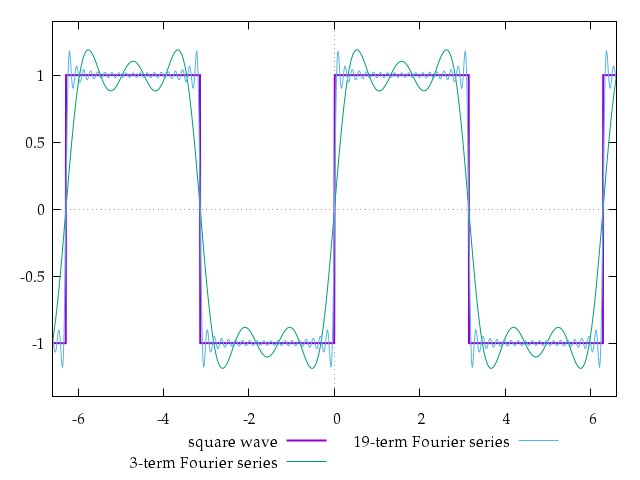# for a clean start, as gnuplot may remember things from previous scripts
reset

# set parameters controlling the appearance of the graphs
set xrange [-2.1*pi:2.1*pi]
set yrange [-1.4:1.4]
set samples 2001
set key below
set zeroaxis

# define the Fourier series' expansion iteratively: sin(x)+sin(3x)/3+sin(5x)/5+...
f(x,n) = (n==1) ? (4/pi)*sin(x) : f(x,n-1) + (4/pi)*sin((2*n-1)*x)/(2*n-1)

# plot the original square wave, and a couple of interesting approximations
set output '/tmp/gnuplot-inline-1520556393.9686322.136613407649.png'
plot sgn(sin(x)) t 'square wave' lw 2,      f(x,3)  t '3-term Fourier series',      f(x,19) t '19-term Fourier series'
unset output

Of course, there are also excellent plotting capabilities within both python, matlab/octave and maple environments, all with much more sophisticated computational capabilities. We will learn these as we go along. However, the flexibility and the ability to generate both screen-friendly bitmap (e.g. PNG) plots and publication-quality scalable vector (SVG, Encapsulated PostScript, LaTeX, etc.) plots makes physica and/or gnuplot useful additions to the scientific toolbox.

## Homework¶

Lord Cavendish was the designer of the original experiment that used a pair of lead spheres and a very sensitive torsional balance to measure the universal gravitational constant, $G$. A manual for a modern version of such a torsional balance is here. As described there, in Fig.18 on p.13, the following set of data was obtained.

Produce a physica or a gnuplot macro that would plot and analyze the data, and calculate $G$ from it. It appears that a good fit would result from fitting $S(t)=S_0+Ae^{-(t-t_0)/\tau}\cos [\omega (t-t_0)]$ to each segment of the experiment with $S_0$, $A$, $t_0$, $\tau$ and $\omega$ the parameters of the fit. Without knowing the exact geometry of the experiment, $\Delta S$ between the two equilibrium positions cannot be used, but perhaps the oscillation period can be extracted and used in the analysis. Use the average of the two values obtained for both sections of the data.

A more careful examination reveals that there might be a small drift in the data. Model it by a small linear-in-time correction term, $\alpha(t-t_0)$, and see if the precision of the fit improves.

The data file is in /work/5P10/Cavendish.dat, and you can execute the next cell to save it to your local file space.

In :
%%file Cavendish.dat
# Cavendish experiment 2012-02-24
## minutes, position_of_dot, cm

# set spheres to one side at 11:45:00, 45 mins since the start
45	57.5
45.5	58
46	58.5
46.5	60.5
47	63
47.5	66
48	69
48.5	72
49	74.5
49.5	76.7
50	78
50.5	78.7
51	78.7
51.5	78
52	76.7
52.5	75.
53	73
53.5	70.8
54	69.2
54.5	67.7
55	66.8
55.5	66.4
56	66.5
56.5	67.1
57	68.1
57.5	69.2
58	70.7
58.75	73.2
59	73.8
59.5	74.8
60	75.6
60.5	76.1
61	76.1
61.5	75.8
62	75.1
62.5	74.2
63	73.2
63.5	72.2
64	71.5
64.5	70.7
65	70.1
65.5	69.9
66	69.9
66.5	70.1
67	70.6
67.5	71.2
68	72
68.5	72.7
69	73.4
69.5	74
70	74.2
70.5	74.6
71	74.7
72	74.1
72.5	73.8
73	73.2
73.5	72.8
74	72.2
74.75	71.8
75	71.6
75.5	71.5
76	71.4
76.5	71.6
77	72
77.75	72.6
78	72.8
78.5	73.1
79	73.5
79.25	73.7
79.5	73.9
79.75	74
80	74.1
80.25	74.1
80.5	74.1
80.75	74.1
81	74.1
81.25	74
81.5	74
81.75	74
82	73.8
82.5	73.7
83	73.4
83.5	73.1
84	72.9
84.5	72.7
85.25	72.7
85.5	72.7
86	72.6
86.5	72.6
87	72.7
87.5	72.8
88	73.1
88.5	73.3
89	73.6
89.5	73.7
90	73.8
90.5	74
91	74
93	73.8
93.5	73.6
94	73.5
98	73.3

# reverse the spheres at 13:25:00, 145 mins since the start
145	75.5
145.5	73.8
146	69.8
146.5	61.5
147	55
147.5	46.5
148	36.5
148.5	31
149	25.8
149.5	22.6
150	21.6
150.5	23
151	26
151.5	30.8
152	36.6
152.5	42.5
153	48.1
153.5	53.2
154	57.3
154.5	59.5
155	60.2
155.5	59.7
155.75	58.8
156	57.7
156.25	56.2
156.5	54.5
156.75	52.8
157	50.8
157.25	48.7
157.5	46.5
157.75	44.5
158	42.5
158.25	40.6
158.5	38.8
158.75	37.2
159	35.9
159.25	34.8
159.5	34
159.75	33.6
160	33.2
160.25	33.2
160.5	33.6
160.75	34.1
161	35
161.25	35.9
161.5	37
161.75	38.2
162	39.5
162.5	42.5
163	45.3
163.5	48
164	50.1
164.5	51.5
165	52.2
165.5	52.1
166	51.2
166.5	49.8
167	48
167.5	45.9
168	43.8
168.5	42
169	40.5
169.5	39.2
170	38.9
170.75	39.2
171	39.7
171.5	40.6
172	41.9
172.5	43.6
173	44.9
173.75	46.9
174	47.5
174.5	48.2
175	48.7
175.5	48.7
176	48.4
176.5	47.7
177	47
177.5	46
178	45
178.5	44
179	43.1
179.5	42.7
180	42.3
180.5	42.1
181	42.5
181.5	42.9
182	43.4
182.5	44.1
183	44.7
183.5	45.5
184	46
184.5	46.4
185	46.7
185.5	46.7
186	46.7
186.5	46.4
187	46.1
187.5	45.6
188	45.2
188.5	44.7
189	44.3
189.5	44.1
190	43.9
191	44
191.5	44.2
192	44.6
192.5	44.7
193	45
193.5	45.4
194	45.7
194.5	45.9
195	46.1
195.5	46.1
196	46.1
196.5	46
197	45.9
197.5	45.6
198	45.4
198.5	45.2
199	44.9
199.5	44.7
200	44.5
202.5	44.6
204	45.4
205	45.7
217.5	45.6
217.75	45.4
218	45.4
218.5	45.3
219	45.3
219.5	45.4
220	45.5

Overwriting Cavendish.dat


These two examples (for gnuplot and physica) are a good start:

In :
%gnuplot inline pngcairo size 640,480 font "Palatino,16"

In :
%%gnuplot
# for a clean start, as gnuplot may remember things from previous scripts
reset

#plot "Cavendish.dat"

#plot "Cavendish.dat" using ($1 < 140 ?$1: NaN ):($2/100) title "forward",\ # "Cavendish.dat" using ($1 > 140 ? $1: NaN ):($2/100) title "reversed"

### requires that TWO blank lines separate data into "blocks" in the data file
plot "Cavendish.dat" index 0 title "forward", "" index 1 title "reverse"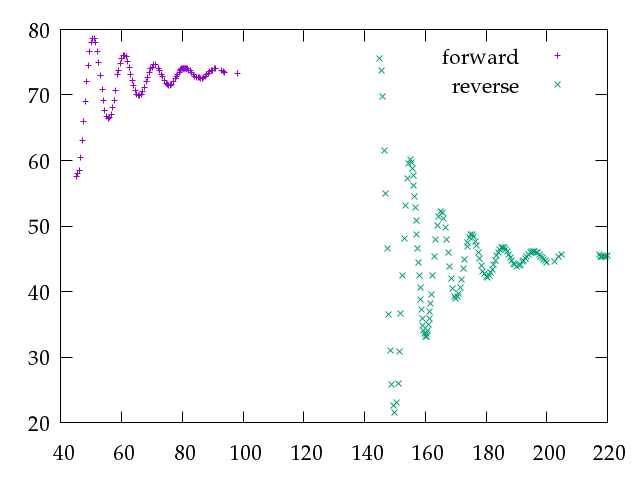# for a clean start, as gnuplot may remember things from previous scripts
reset

#plot "Cavendish.dat"

#plot "Cavendish.dat" using ($1 < 140 ?$1: NaN ):($2/100) title "forward", # "Cavendish.dat" using ($1 > 140 ? $1: NaN ):($2/100) title "reversed"

### requires that TWO blank lines separate data into "blocks" in the data file
set output '/tmp/gnuplot-inline-1520556394.1015484.651333514107.png'
plot "Cavendish.dat" index 0 title "forward", "" index 1 title "reverse"
unset output

The data is in and is separated into two parts. Complete this homework by adding the fit command, which might look like this, if the dependence were a purely linear function:

    set dummy t
S(t)=S0+alpha*t
S0=73
alpha=1
fit "/work/5P10/Cavendish.dat" index 0 via S0,alpha


Do not skip the step of setting reasonable initial values for each fit parameters, and do not use the initial values of zero, as the fit command chokes on those. By a happy coincidence, an old gnuplot tutorial demonstrates how to do just what we need to do: https://www.cs.hmc.edu/~vrable/gnuplot/using-gnuplot.html

The same, of course, can be done in physica (which is perfectly happy to start with a zero estimate for parameters, and defaults them to 1.0 if not specified):

    read\format\errskip /work/5P10/Cavendish.dat (*) t,x
scalar\vary x0,alpha
x0=73
alpha=0
fit\weight (t<140) x=x0+alpha*t